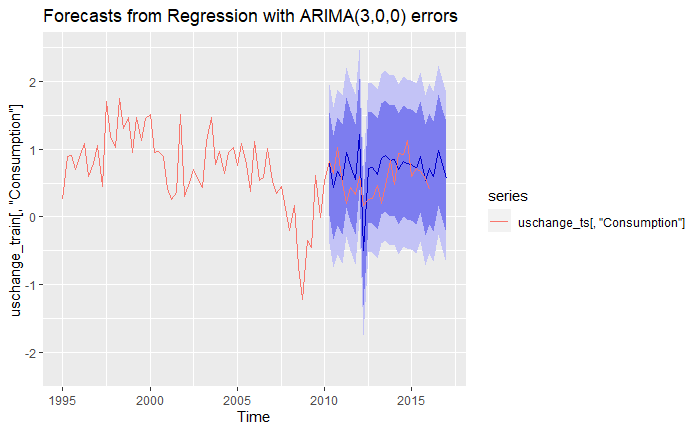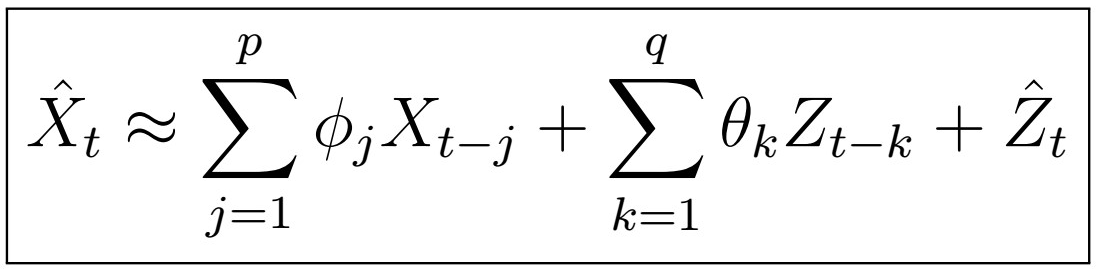# A Complete Introduction To Time Series Analysis (with R):: Exogenous models# A Complete Introduction To Time Series Analysis (with R):: Estimation of ARMA(p,q) Coefficients (Part II)The ARMA(p,q) model implies that X_{t} can be expressed in the form above.

# A Complete Introduction To Time Series Analysis (with R):: Estimation of ARMA(p,q) Coefficients (Part I)